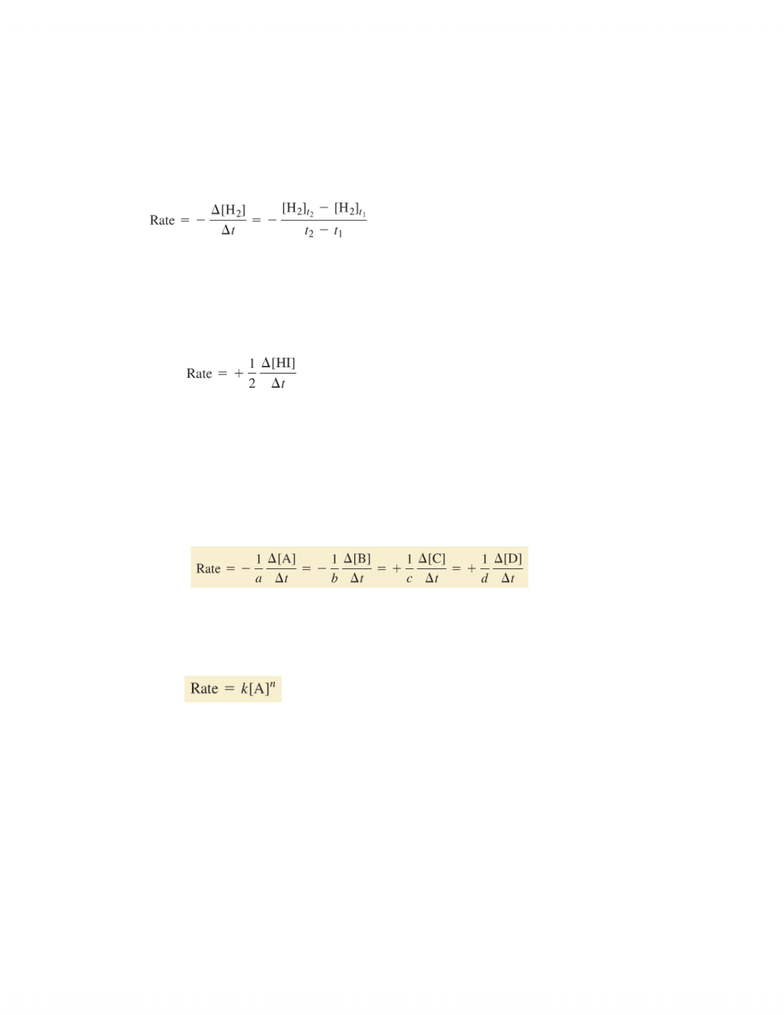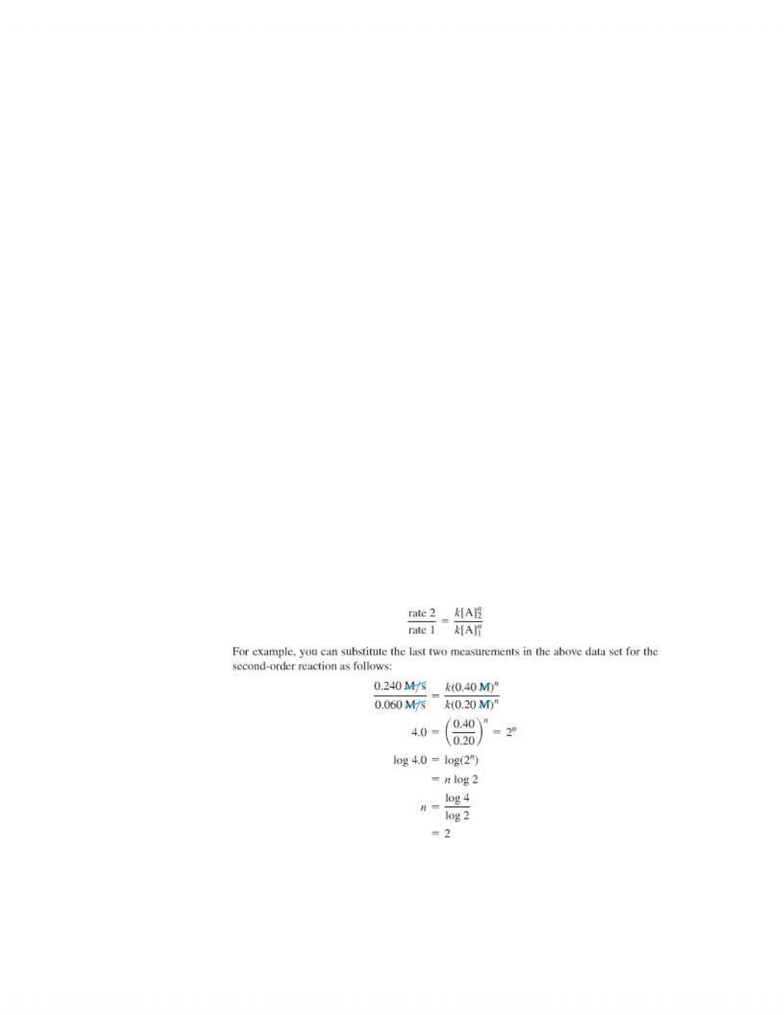Study Guides (400,000)
CA (160,000)
UTSC (10,000)
Chemistry (200)
CHMA11H3 (100)

# CHMA11H3 Study Guide - Activation Energy

This preview shows pages 1-2. to view the full 7 pages of the document.Chapter 13: Chemical Kinetics
The rate of a chemical reaction is a measure of how fast the reaction occurs
(concentration over time):
The rate can also be defined with respect to the product of the reaction. Because
product concentrations increase as reaction proceeds, the change in concentration of
a product is positive.
The factor (1/2) in this definition is related to the stoichiometry of the reaction.
As the reactants transform to products, their concentrations decrease, and the
reaction slows down.
Average and instantaneous rates of change can be calculated using a chart or graph
Rate Law: A relationship between the rate of a reaction and the concentration of
the reactants
k is a constant of proportionality called the rate constant and n is the reaction
order
The value of n determines how the rate depends on the concentration of A
First-Order Reaction:
oin a first-order reaction, the rate of the reaction is directly proportional to the
concentration of the react:
www.notesolution.com

Only pages 1-2 are available for preview. Some parts have been intentionally blurred.Rate = k[A]1
othe rate slows down as the reaction proceeds because the concentration of the
reactant decreases
second-order reaction:
oin a second-order reaction, the rate of the reaction is proportional to the
square of the concentration of the reactant:
Rate=k[A]2
othe rate is more sensitive to the reactant concentration (than in first-order)
Determining the Order of a Reaction:
oThe order of a reaction can be determined only by experiment. A common way
to determine reaction order is by the method of initial rates measured at
several different initial concentrations
oEx: when the concentration of a reactant doubles, as well as the rate, the
initial rate is directly proportional to the ignition concentration, making it a
first-order reaction.
oAnother way is substituting any two initial concentrations and the
corresponding initial rates into a ratio of the rate laws to determining the
order (n):
Reaction order for multiple Reactants:
www.notesolution.com